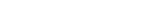MathExamle.com# Addition and Subtraction of decimals

Create worksheets of mathematical examples with addition and subtraction of decimals, select type of a generator and viewing some mathematical examples

### Partitive

Creates examples with addition and subtraction operations of decimals, the answers are always positive

3 Variants 4 Levels
 44.5-2= 33.6+13+53.4= 49.5-(11.9+33.2)= 67.7-6.1-41.9=
Variant: A Level: Medium

Creates examples with addition and subtraction operations of decimals, the answers can be negative

3 Variants 4 Levels
 -84-14.4= 5.9+73.4= 15.4-65= -87.1-7= -79.3+7.8= -1.3-54.2=
Variant: 1 Level: Medium